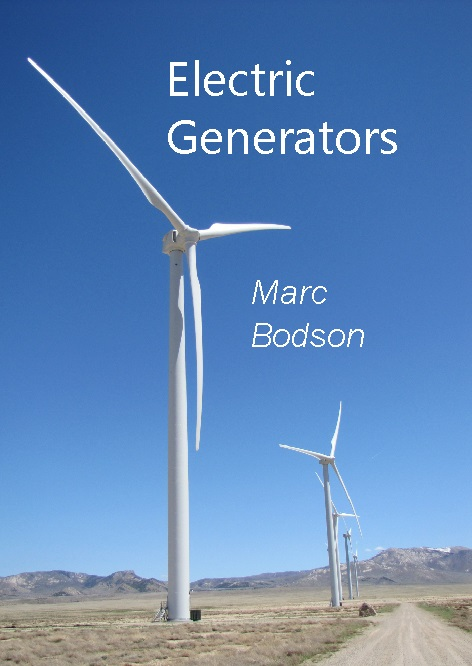Electric GeneratorsTo be published through Amazon, expected for end of 2022.ISBN: 979-8542666211.

Software labs

Experiments are performed using a variety of models in Matlab/Simulink.

Lab 1:   Introduction to Simulink with an RL circuit
(Problem 1.2)
Lab 2:   Wind power generation (Problems 1.3 and 1.4)
Lab 3:   DC generator (Problem 2.4)
Lab 4:   Self-excited DC generator (Problem 2.6)
Lab 5:   Single-phase AC power (Problem 3.1)
Lab 6:   Permanent-magnet synchronous generator (Problem 4.3)
Lab 7:   Squirrel-cage induction generator (Problem 5.4)
Lab 8:   Self-excited induction generator (Problem 5.5)
Lab 9:   Wound-field synchronous generator (Problem 6.4)
Lab 10:  Control of three-phase inverter (Problem 7.1)
Lab 11:  Doubly-fed induction generator (Problem 8.1)
Lab 12:  Dynamics of a wound-field synchronous generator (Problems 9.1 and 9.2)

Simulink tutorial using Lab 1 (intro1.mp4) (intro2.mp4) (intro3.mp4)

Simulation models

Blank simulation model: blank.mdl
Collection of Simulink blocks to simulate electric generators: generator_blocks.mdl
Wind turbine simulation:
wind_turbine.mdl. Wind data file: wind.mat

Free reference for basics of systems and control

Textbook: Foundations of Control Engineering, available at: https://www.ece.utah.edu/~bodson/ifs/

This web page is maintained by Professor Marc Bodson, of the Department of Electrical and Computer Engineering at the University of Utah. Please see the University of Utah Web disclaimer. For questions or comments, please send an e-mail to marc.bodson@utah.edu.

Last updated: July 19, 2022.